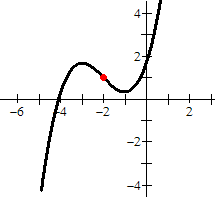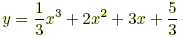# point of symmetry

Point of Symmetry

A special center point for certain kinds of symmetricfigures or graphs. If a figure or graph can be rotated 180° about a point P and end up looking identical to the original, then P is a point of symmetry.

 Example:This is a graph of the curvetogether with its point of symmetry (–2, 1).The point of symmetry is marked in red.